# Homeomorphism group

The group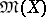of homeomorphic mappings of a topological spaceonto itself (cf. also Homeomorphism). Ifis a compact manifold, then the algebraic properties of, especially the structure of its normal subgroups, determineup to a homeomorphism . In particular, forit is known that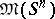is a simple group. This is also true of the Cantor set, the Menger curve, the Sierpiński curve, and the sets of rational or irrational points on the real line . For a manifoldthe minimal normal subgroup inis the subgroup generated by the homeomorphisms that are the identity outside domains in.

The groupmay be topologized in different manners (cf. Space of mappings, topological). Of fundamental importance are the compact-open topology and (ifis metrizable) the fine-topology, in which neighbourhoods of the identityare defined by strictly-positive functions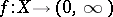, and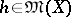forms part of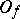if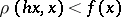for all, whereis the metric in. However,need not necessarily be a topological group in these topologies, since the mapping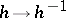is not always continuous, and even if it is,need not be a topological group of transformations (cf. also Transformation group), i.e. the mapping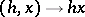can be discontinuous . However, ifis a manifold, thenis a topological group of transformations in both these topologies. The study of the topological properties ofis of interest, in the first place, for a homogeneous space, i.e. such that the action ofonis transitive. However, the available studies are far from complete even for simple manifolds. Thus, it is not known (1977) ifis an infinite-dimensional manifold, even though it is (for a metrizable manifold) locally contractible in the fine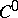topology . In particular, two sufficiently-close homeomorphisms can be connected by an isotopy (cf. Isotopy (in topology)). For an open manifold which is the interior of a compact manifold this is also true in the compact-open topology.

The quotient group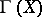ofby the component of the identity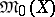is called the homeotopy group of. Generally speaking,is not identical with the group of homeomorphisms that are homotopic to the identity, but they coincide for two-dimensional and some three-dimensional manifolds (e.g. for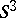,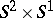, etc.). The homotopy properties of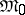have been studied for two-dimensional manifolds; this proved useful in establishing homological properties of braid groups (cf. Braid theory).

Of special importance in the theory of manifolds is the study of certain subgroups of the group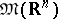, e.g. of the subgroup of diffeomorphisms. This study is made more difficult by the fact that the subgroups are not closed, while the topology of quotient spaces is unsatisfactory. For this reason one considers the semi-simplicial groups (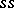-groups)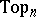, in which the-dimensional simplexes are the fibred homeomorphisms of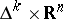that are stationary on the zero section (here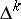is the standard-simplex). The boundary homeomorphisms and degenerations are defined with the aid of the standard mappings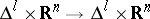. The-monoidsof the-groups,,(homotopy equivalences of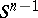of piecewise-linear, smooth and orthogonal mappings of) are defined in the same manner, and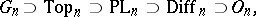and the quotients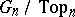, etc., have the natural structures of-complexes, which makes it possible to study homotopy properties of these imbeddings.

The study of the various subgroups of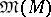for manifoldsforms the subject of several disciplines. In particular, the study of homeomorphism groups which preserve certain structures belongs to the corresponding branches of mathematics. Of considerable interest are algebraic problems connected with automorphism groups of trees and other graphs.